Courses

# Test: Basic Concept- Thermodynamics

## 20 Questions MCQ Test Chemistry for JEE | Test: Basic Concept- Thermodynamics

Description
This mock test of Test: Basic Concept- Thermodynamics for JEE helps you for every JEE entrance exam. This contains 20 Multiple Choice Questions for JEE Test: Basic Concept- Thermodynamics (mcq) to study with solutions a complete question bank. The solved questions answers in this Test: Basic Concept- Thermodynamics quiz give you a good mix of easy questions and tough questions. JEE students definitely take this Test: Basic Concept- Thermodynamics exercise for a better result in the exam. You can find other Test: Basic Concept- Thermodynamics extra questions, long questions & short questions for JEE on EduRev as well by searching above.
QUESTION: 1

### Direction (Q. Nos. 1-15) This section contains 15 multiple choice questions. Each question has four choices (a), (b), (c) and (d), out of which ONLY ONE option is correct. Q.  Assign the sign of work done (based on SI convention) in the following chemical changes taking place against external atmospheric pressure :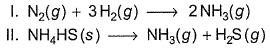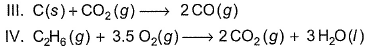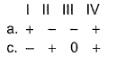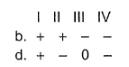Solution:

Work done = - (change in moles) RT For work done positive change in moles negative and vice versa We only cosider the moles that are in gass phase.

QUESTION: 2

### For the following process, H2 (g) → 2 H(g), it absorbs 436 kJ mol-1. Thus,

Solution:

In this process, one molecule of hydrogen gas dissociated into two gaseous atoms i.e. the number of gaseous particles increase leading to  a more disordered state.
.Breaking of bond requires energy

It is not mentioned that the process is isobaric. If we assume that process is isobaric because volume can not remain constant as number of gaseous particles are increased, then change in internal energy = 436 kJ mol-1. The data of absorption gives us the value of q.

QUESTION: 3

### Suppose the elements X and Y combine to form two compounds XY2 and X3Y2. When 0.1 mole of XY2 weighs 10 g and 0.05 mole of X3Y2 weighs 9 g, the atomic weights of X and Y are

Solution:

Let atomic weight of x = Mx
atomic weight of y = M

we know,
mole = weight /atomic weight
a/c to question,

mole of xy2 = 0.1
so,
0.1 = 10g/( Mx +2My

Mx + 2My = 100g -------(1)

for x3y2 ; mole of x3y2 = 0.05

0.05 = 9/( 3Mx + 2My

3Mx + 2My = 9/0.05 = 9 × 20 = 180 g ---(2)
solve eqns (1) and (2)

2Mx = 80
Mx = 40g/mol

and My = 30g/mole

QUESTION: 4

Five moles of an ideal gas at 27° C are allowed to expand isothermally from an initial pressure of 10.0 atm to a final pressure of 4.0 atm against a constant external pressure of 1.0 atm. Thus, work done will be

Solution:

Work done during isothermal irreversible process is given by the formula w = -pext (nrt/p2-nrt/p1)

QUESTION: 5

56 g of iron reacts with dilute H2SO4 at 27° C . W ork done (in cals) in

I. closed vessel of fixed volume and
II. an open vessel is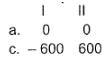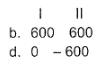Solution:

Use ∆H=∆U+∆NgRT FOR 1. we need to find work done in constant volume that is ∆U which will be 0 as it is nCv∆T and since ∆T is zero so ∆U is zero .
For 2. we need to Find ∆H =∆U+∆NgRT Since ∆U is zero so∆H=∆NgRT
R=2 T =300and ∆Ng is = -1

QUESTION: 6

Consider the following properties.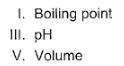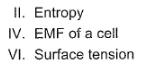Select intensive and extensive properties.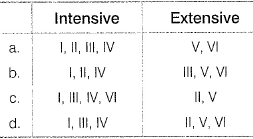Solution:

Intensive means that the property is mass independent. We can see that Boiling point, pH, EMF of cell and surface tension are intensive pont .
While Extensive property  depends on mass and in given options Volume and entropy are mass dependents.

QUESTION: 7

Consider the following properties.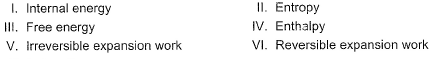State functions are

[IITJEE2009]

Solution:

First 4 are fundamental examples of state functions as they are path independent. Since reversible expansion depends on the path followed by process, so it is a path function. However, irreversible expansion work is independent of the path and so, it is a state function.

QUESTION: 8

For an endothermic reaction when ΔH represents the enthalpy of the reaction in kJ mol-1, the minimum value for the energy of activation will be

[IIT JEE 1992]

Solution:

In endothermic reactions, the energy of reactants is less than that of the products. Potential energy diagram for endothermic reactions is,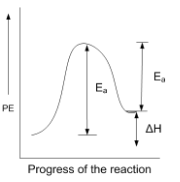Where Ea = activation energy of forwarding reaction

Ea' = activation energy of backwards reaction

ΔH = enthalpy of the reaction

From the above diagram,

Ea = Ea' + ΔH

Thus, Ea > ΔH

QUESTION: 9

One mole of an ideal gas is put through a series of changes as shown in the figure in which 1,2,3 mark the three stages of the system. Pressure at the stages 1, 2, and 3 respectively will be (in bar)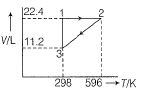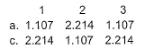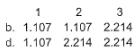Solution:

Applying ideal gas eqn. at 1
p×22.4 = 1×0.0821×298        (since vol. Is in L. R should haave constaant with lit in it.)
p = 1.09 atm. To convert it into bar, we multiply it by 1.01 or we have 1.03 bar
Similarly at 2 and 3, we get value of pressure at 2 and 3.
The most closest answer is option d

QUESTION: 10

An ideal gas expands in volume from 1 × 10–3m3 to 1 × 10–2m3 at 300K against a constant pressure of 1 × 105Nm–2. The work done is

[AIEEE 2004]

Solution: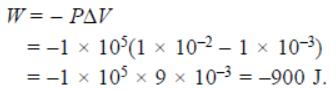QUESTION: 11

One mole each of CaC2, AI4C3 and Mg2C3 reacts with H2O in separate open flasks at 25° C. Numerical value of the work done by the system is in order

Solution:

i) CaC2 + 2H2O  →  C2H2(g) + Ca(OH)2
ii) Mg2C3 + 4H2O → CH3-C≡CH(g) + 2Mg(OH)2
iii) Al4C3 + 12H2O → 3CH4(g) + 4Al(OH)3
Work done = -∆ngRT
Or W ∝ ∆ng
Wecan see that in case iii) we have maximum no of moles in the product side(∆ng = 3). So work done will be maximum in case iii). After that in i) and ii), we have the same number of moles on the product side(∆ng = 1), so work done will be the same.
Therefore, option c is correct.

QUESTION: 12

Bond energy of (N— H)bond is x kJ mol-1 under standard state. Thus, change in internal energy in the following process is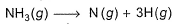Solution:

The correct answer is Option B.

H−N−H (three N-H) bonds
∣
H
Thus, ΔE=3xKJmol-1

QUESTION: 13

Temperature of one mole of helium gas is increased by 2°. Thus, increase in internal energy is

Solution:

∆U = nfRT/2
Since He is monoatomic, so f(degree of freedom) = 3
∆U = 3×1×2×2/2   (We have taken R = 2 cal K-1 mol -1)
= 6 cal

QUESTION: 14

The internal energy change when a system goes from state A to B is 40kJ/mole. If the system goes from A to B by a reversible path and returns to state A by an irreversible path what would be the net change in internal energy?

Solution: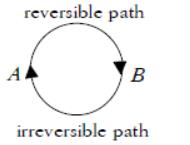We know that for a cyclic process the net change in internal energy is equal to zero and change in the internal energy does not depend on the path by which the final state is reached.

QUESTION: 15

The density of ice at 0°C is 0.915 g cm-3 and that of liquid water at 0°C is 1.0 g cm-3. Work done for melting 1 mole of ice at 1.00 bar is

Solution: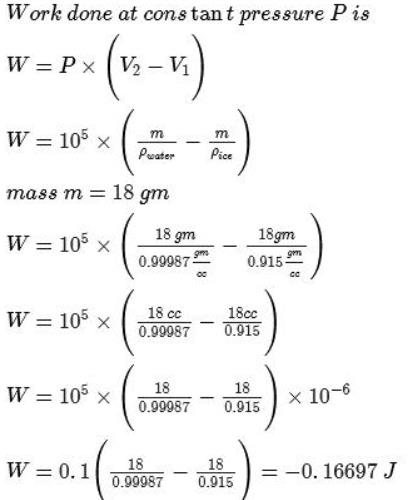*Answer can only contain numeric values
QUESTION: 16

Direction (Q. Nos. 16 - 18) This section contains 3 questions. when worked out will result in an integer from 0 to 9 (both inclusive).

Q. Temperature of one mole of an ideal gas is increased by 1° at constant pressure. What is mechanical work done (in cals)?

Solution:

From ideal gas equation
PV=nRT
hence PV=W
W = nR∆T
W = 1×2×1 = 2cal

*Answer can only contain numeric values
QUESTION: 17

A sample of gas changes from p1 V1 and T1, to p2, V2 and T2 by one path and then back to  and T1, by another path. How many of the following must be zero for the gas in this cycle?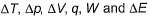Solution:

∆T, ∆p, ∆V and ∆U = 0 (also they are state function)
∆U = q+w. So, q and w are not zero.
Hence we have 4 parameters equal to zero after the whole process.

*Answer can only contain numeric values
QUESTION: 18

What will be the temperature change of 1.00 mole of CH3OH(g) if 100 J of heat is added to it under constant volume conditions?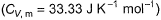Solution:

For heat added at constant volume, Work doe = 0
∆U = T1T2 nCv,mdT
Or ∆U = nCv,m∆T
∆T = ∆U/nCv,m = 100/1×33.33 = 3
So, the correct answer is 3

QUESTION: 19

Direction (Q. Nos. 19-20) This section contains a paragraph, wach describing theory, experiments, data etc. three Questions related to paragraph have been given.Each question have only one correct answer among the four given options (a),(b),(c),(d).

The following diagram represents the (p-V) changes of a gas.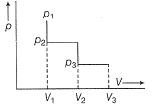Select isochoric changes

Solution:

Isochoric change is one in which volume doesn't change(remains constant) From P1 to P2 , volume is constant(V1) (also work done = 0)

QUESTION: 20

The following diagram represents the (p-V) changes of a gas.Total work done is

Solution:

Work done is the area enclosed by PV graph with volume axis.